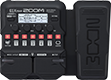# Metallica NEM humbucker

Discussion in 'Zoom G1/G1X Four' started by Edu Cardoso, Jul 1, 2023.

1. Metallica NEM humbuckerDevice: Zoom G1X Four
Firmware: 2.00

Name on device: MET-NEM
Optimized for: Phones/Speaker

Effects chain:Use Humbucker first position with fingers

Effect: "Comp" (Dynamics), active - "yes"
"Sense" = 1
"Attack" = Fast
"Tone" = 10
"Volume" = 100

Effect: "Aco.Sim" (Overdrive / Distortion), active - "yes"
"Top" = 80
"Body" = 50
"Tone" = 50
"Volume" = 100

Effect: "Chorus" (Modulation), active - "yes"
"Depth" = 20
"Rate" = 25
"Tone" = 5
"Mix" = 50

Effect: "Hall" (Reverb), active - "yes"
"PreD" = 50
"Decay" = 10
"Mix" = 70
"Tail" = On

Effect: "Gt GEQ 7" (Filter), active - "yes"
"100" = 3.0
"200" = 0.5
"400" = -5.0
"800" = -2.5
"1.6k" = -5.5
"3.2k" = 6.5
"6.4k" = 8.0
"VOL" = 100

Patch Volume: 100